# Indifference curve

Classified in Economy

Written at onEnglish with a size of 561.51 KB.

No. 5 YEARS TO DEVELOP IN CLASS
THEORY OF INDIFFERENCE CURVES
Prof. Thomas S. Alvarez

ECONOMICS AND INDUSTRIAL ORGANIZATION I

INDIFFERENCE CURVES

Table below shows 1.La points in four different curves for a consumer indifference.
(a) Draw the indifference curves I, II, III and IV in the same system of axes.
(b) What are the indifference curves?

IIIIIIIV QxQyQxQyQxQyQxQy21331251271236485, 598944,556,368,39753,56577106,36374,486115,772,78495,4125,3
a)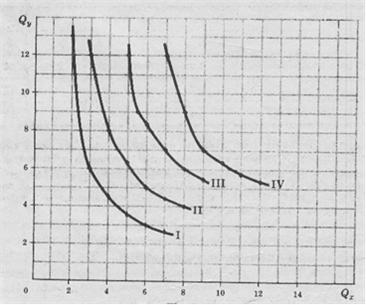b) Indifference curves graphically display the tastes and preferences of consumers (in the analysis of utility, total utility curve introduced consumer tastes). The consumer is indifferent as to all the various combinations of X and Y on the same indifference curve but rather points higher at a curve in a lower points. While we have chosen to represent the tastes of a consumer drawing only three or four indifference curves,the field of such curves is dense, ie there are an infinite number of them. All indifference curves of a consumer give us the consumer's indifference map. Different consumers have different indifference maps. When changing tastes of an individual, his indifference map changed.
2. (A) Does it require cardinal measure of utility or satisfaction to draw a set of indifference curves?
(b) What are the characteristics of the indifference curves?
(a) To draw a set of indifference curves of order or just need a range of consumer preferences. A cardinal measure of utility or satisfaction is not necessary or specified. That is, we need not know either the absolute amount of utility that a consumer receives for being in a specific curve of indifference, or to what extent its value increases when going to a higher curve. All you need to know to get the indifference curves of a consumer, is whether this consumer is indifferent, if you prefer or do not prefer each combination of X and Y combinations.

(b) Indifference curves slope downward, are convex to the origin and do not cross each other. There must be, and generally are not parallel.

3. (A) Find the TMSxy among all the four consecutive points indifference curves in Problem 1.
(b) What is the difference between TMSxy and UMgx?
(a) IIIIIIIVQxQyTMSxyQxQyTMSxyQxQyTMSxyQxQyTMSxy213 - 312 - 512 - 712-3674845, 59689344,51,556,31,768,31,497253,51651,3771,3106,30,7630,574,40,6861115,70,672,70,3840,495 , 40,6125,30,4
(b) measures the amount of TMSxy And a consumer is willing to deliver for an additional unit of X (and stay on the same indifference curve). That is, TMSxy = - (: Q /? Qx). The UMgx measures the change in total utility a consumer receives when modifying their consumption by one unit of X. That is, UMgx =? UTX, / Qx. By measuring the TMSxy, both X and Y vary. By measuring the amount of Y UMgx (among others) is kept constant. Thus the measured TMSxy distinct from UMgx.

4. In the same set of axes, draw three indifference curves showing perfect substitutability between X and Y.
For X and Y are perfect substitutes, TMS,, must be constant. This means that whatever the indifference curve that we are, and any point of it where we are, we have to sacrifice the same amount of Y to obtain an additional unit of X. For example, going from point A to point B on indifference curve III, TMSxy equals 2. Similarly, going from B to C the TMSxy also equals 2. If the indifference curves at length had a slope of -1 (so that TMSxy = 1), X and Y not only be perfect substitutes but that might be considered as the same product from the standpoint of the consumer. For example, X and Y could be two beer brands and the consumer is indifferent to drink either. Q 8 A 6 B 4 C 2 IIIIII0246Qx
5. In the same set of axes, draw three indifference curves to show that X and Y are perfectly complementary.

For X and Y are perfect complements, the TMSxy and TMSyx, must be both equal to 0. For example, the points D, E and F are all in the indifference curve I, and yet the point F includes the same amount of X over Y, but the point E. Thus, the consumer is saturated and TMSxy X equals 0. Similarly, Section D contains the same amount of X, but more of Y than point E. The consumer is saturated with Y, and TMSyx = 0. Automobile and gasoline can be regarded as perfect complements. In general, the indifference curves are not straight lines and right angles but they show some curvature. The closer the shape of an indifference curve! Inea line, the greater the degree of possible substitution between X and Y. III Q 864Qx 2 III 0246
6.En the same set of axes, draw three curves that show an increasing indifference TMSxy as we descend along the curves.

The indifference curves are concave to the origin and thus show that TMSxy increases as we descend along the curves. For example, going from point G to point H on curve III, the TMSxy = 1. In going from H to J, the TMS.xy = 2. In Problem 13 we will study the effect that such indifference curve is for consumer equilibrium. Q 4 GH 2 J I 0246 IIIII Qx

BUDGET RESTRICTION LINES

7. Suppose the price of a product and is \$ 1 per unit, while the price of X is \$ 2 per unit, and that individual's money income is \$ 16 per unit time and spends it all in X and Y.
(a) Draw the budget constraint line for this consumer, and
(b) Explain the reason for the shape and properties of the budget constraint line in part (a).

a)
Q (b) If the consumer spends all his income on the product and would buy 16 units. If you spent it all on the product X could buy 8 units. Uniting these two points with a straight line, we get the line consumer budget constraint. This line gives us all the different combinations of X and Y that the consumer can buy. Thus, one could buy 16Y and 8X, 14Y and 1X, 2X and 12Y ... 0Y and 8X. Note that for every 2 units of Y that sacrifice, you can buy an additional unit of X. The slope of the budget line has a value of -2 and remains constant. Should also be noted that all budget line items show that consumers are spending all their income in X and Y. This means that your PxQx + Py Q = M = \$ 16.161514131211109876543210 123456789 101112 Qx
8.Dados consumer money income (M), Py and Px:
a) indicate the amount of Y could afford if all their income spent on Y,
b) indicate the amount of X if he could buy all of their income spent on X,
c) find the slope of the budget restriction in terms of Px and Py, and
d) find the general equation of the line of budgetary restraint.

AbcQyQ y0 Q MQ y0 = x0 Qx Qx ------ when Py = 0 MQ = ------- x0 when Q = 0 Px? YQ y0 Slope = ----- = - ---- - =? XQ x0 M / M Px Py Px = - ------- = - ----- x ----- = ---- M / M Px Py Py
d) The general equation of a straight line can be written as y = a + bx, where a = y-intercept value of I when x = 0, b = slope of the line. For the answer to part (a) we know that a = M / Py for the answer to part (c) we know that b = -Px/Py. Therefore, the general equation of the line budget constraint is
M Px
Q = ------ - ------ Qx
Py Py
Multiplying each term in the above equation by P and then rearranging terms, we obtain an equivalent way of expressing the equation of the line of budgetary restraint. That is,

M Px
(Py) (Q = ------ - ------ Qx) becomes Q = M Py - Px Qx
Py Py

Transposing the last term (-PxQx) to the left of the equal sign, we obtain PxQx + PyQy = M

9. (A) Find the equation line specific budget constraint in Problem 7.
(b) Show an equivalent way of expressing the specific equation of part (a).

(a) In Problem 7, the y-intercept (a) = M / Py = \$ 16 / \$ 1 = 16. The slope of the budget line (b) = -Px/Py = -2 / 1 = -2. Therefore, the equation specific budget line in Problem 7 gives Q = 16-2QX. Substituting various values of Qx in the equation, we obtain the corresponding values of Q. So when Qx = 0, Q = 16; when Qx = 1, Q = 14, where Qx = 2, Q = 12, ... ... where Qx = 8, Q = 0.

(a) Another way to write the budget line equation in Problem 7 is
(\$ 2) (Qx) + (\$ 1) (Q) = \$ 16

Substituting various quantities of a product in this equation, we obtain the corresponding quantities of another product that the consumer must purchase in order to remain in their budget line. For example, if Qx = 2, the consumer has to buy 12 units of Y to stay in their budget line (ie if you spend all your income from \$ 16 in X and Y).

CONSUMER EQUILIBRIUM

10. If consumer tastes the indifference curves given in Problem 1 and its income and price restrictions gives them the budget line for Problem 7.
(a) find geometrically the point where the consumer is in equilibrium, and
(b) Explain why this is an equilibrium point, what is true of the slope of the indifference curve and budget line in equilibrium?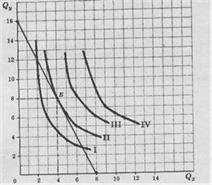(b) The consumer is in equilibrium at point E, in which its budget line is tangent to his indifference curve II. The indifference curve II is the highest it can reach, given their budget line. How are tangent, the absolute slope of the indifference curve II (TMSxy) and the absolute slope of the budget line (Px / Py) are equal at point E. That is, at point E TMSxy = Px / Py = 2. Because the field of indifference curves or indifference map is dense, is assured a point of tangency (and balance of the consumer). 11. (A) Explain why the points G, D, C and F ( that is the same as in Problem 10) are not equilibrium points of the consumer.
(b) Explain in terms of the slopes of indifference curves and slopes of the budget line by which a movement from point C to point E increases consumer satisfaction and
(c) Do the same for a move from F to E.

(a) Given the prices of X and Y, the consumer's income is not sufficient to reach the G spot in the indifference curve III. At point D, the consumer is on the indifference curve I and not spending all their income. At points C and F would be spending all their income but is still in the indifference curve I, and therefore is not maximizing their satisfaction.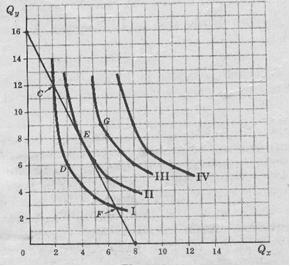(b) At point C, the absolute slope of the indifference curve I (indicating what the consumer is willing to do) exceeds the absolute slope of the budget line (which indicates what you can do in the market). That is, starting at point C the consumer is willing to give up more than 6 units of Y to obtain 1 additional unit of X remaining at the same indifference curve I. However, you can get an additional unit of X in the market giving up only 2 units of Y. So down its line from point C to point E, increases consumer satisfaction.
(c) At point E the absolute slope of the budget line is greater than the absolute slope of the indifference curve I. This means consumers can get more of the market and what it is willing to accept to give up 1 unit of X. Thus, bringing its budget line from point F to point E, increases consumer satisfaction. At point E, the TMSxy = Px / Py.

12. (A) To express mathematically the equilibrium condition on the theory of consumer indifference curve.
(b) Show that if there is a cardinal measure of utility, the condition (a) reduces to
UMgx UMgy
--------- = ---------- With PxQx + PyQy = M
Px Py

(a) As shown in Problem 8 consumer budget line equation gives PxQx + PyQy = M. At the point that this line is tangent to an indifference curve, the slope of the curve TMSxy absolute equals the absolute slope of budget line Px / Py (see Problem 10). Therefore TMSxy = Px / Py with PxQx + PyQy = M is the equilibrium condition on the theory of indifference curves.
(b) Suppose that a consumer can measure profit (and therefore the marginal utilities) and numerically for some Qx, and Qy, UMgx = 5 utils and util UMgy = 1. Then the consumer would be willing to give a.5 units of Y in exchange for an additional unit dex, because the business would not change its net income. So TMSxy = 5 for the given quantities, and generally TMSxy = UMgx / UMgy. Substituting this expression the left side of the equation in part (a), we obtain
UMgx Px UMgx UMgy
--------- Or ---------- = -------- = -----------
UMgy Px Py Py

13 Draw a diagram showing that

(a) if the indifference curves are convex to the origin, but everywhere are flatter than the budget line, the consumer maximizes satisfaction if you use only the product Y,
(b) If the indifference curves are convex to the origin but are everywhere steeper than the budget line, the consumer maximizes satisfaction if you eat only product X, and
(c) If the indifference curves are concave to the origin, the consumer maximizes his satisfaction whether the product consumes only X or only the product Y.
(d) Would you expect that the indifference curves have any of these ways in the real world? Why?

Table (a) the indifference curve II is the highest it can reach the consumer budget line DF. To reach it (and therefore to be in equilibrium) the consumer has to spend all their income in the product Y (ie, OD units should buy Y and no X). The fact that the area is dense indifference curves always ensures a point of balance on the Y axis
Table (b) the consumer is in equilibrium when you spend all your income for purchase of Units of X (and no Y).
Table (c) City budget line is tangent to the indifference curve I at point K. However, this is not a point at which consumers are maximizing their satisfaction, since it could reach the indifference curve II to consume only Y (D).

(d) In the real world, the consumer does not spend all their income in a single product, therefore the indifference curves are as shown in Tables (a), (b) and (c).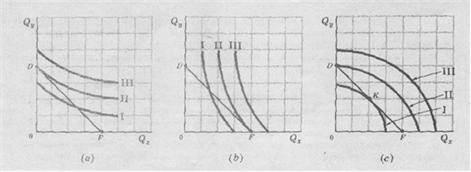EXCHANGE

14. Suppose individuals A and B each have between them a combined total of 14 units and 16 units of X. Assume further that the likes of A are represented by indifference curves I, II and III, while the likes of B are represented by indifference curves I ', II', lll '(with origin at 0'). (In fact, what we have done here is to rotate the set of indifference curves B 180 degrees and place on top of the figure of indifference curves of A, in a way that the ring formed has the dimensions specified 14Y and 16X)
(a) What does each point inside the ring?
(b) Is there a basis for mutually beneficial exchange between A and B to point C? Explain.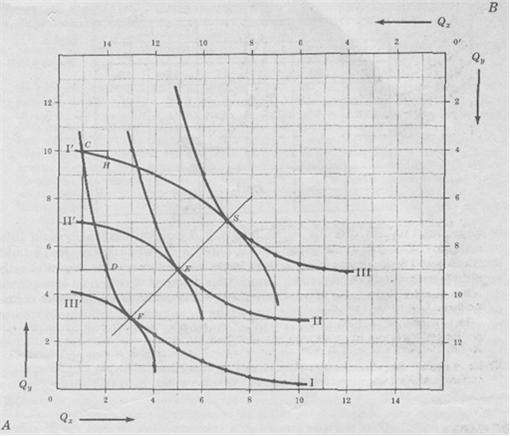(a) Any point inside the ring (or on it) represents a particular distribution of 14Y and 16X between individuals A and B. For example, point C indicates that A has 10Y and 1 while B has 4Y X and 15 X.

(b) Since the point C TMgSxy A exceeds the individual's TMgSxy of individual B, there is a basis for mutually beneficial exchange between A and B. Starting in C, individual A would be willing to part with 5 And for an additional unit of X (and then move to point D on indifference curve I). Individual B would give up to 1X in exchange for 0.4 of Y (and then move from point C to point H on the indifference curve I '). Since A is willing to hand over and what is necessary to induce B to give up one unit of X, there is no basis for exchange. In this exchange, handing over her And in return you give 1X B.

15 .-. Explain what happens if, starting at point C of Table
(a) the individual to change 3Y by 6X with individual B,
(b) the individual 2X per 7Y B changes with the individual A, and
(c) the individual to change 5Y by the individual 4X B.

(a) If A to B 3Y give in exchange for 6X, A would move the point C on his indifference curve I to S of his indifference curve III, while B would move along his indifference curve I 'from C to S. To get the full benefit of the exchange, while B is not win or lose anything (since B is in the same indifference curve I '). At point S, the indifference curves III and I 'are tangent, so that their slopes are equal. This means that at that point the TMgSxy of A is equal to B, so there is no basis for further exchanges. (From point S, the amount of Y that A would be willing to provide for a unit of X is not sufficient to induce B to divest a unit of X).

(b) If B to A 2X surrender in exchange for 7Y, individual B would drop from point C on his indifference curve I 'to point F on his indifference curve III'. In this case, all profits from this exchange would be for B. Individual A would gain or lose anything, since he would remain in the same indifference curve I. At F, the TMgSxy of A is equal to B, so there is no basis for further exchanges.

(c) Starting at point C on indifference curves I and I ', if the individual A changes with B 5Y by 4X (and moves to point E), both A and B benefit since the point E is on curves different from indifference, II and II '. Connecting points of contact for the indifference curves of A and B obtain the contract curve FS. When A and B are not on the contract curve, A or B or both may gain from the exchange. When both are on the contract curve, it is not possible to make more profit with the exchange.

16.-Suppose that the likes of A are represented by indifference curves I, II and III in case 1, while the likes of B's indifference curves represent the table below.
Assume further that individuals A and B each have between them a total of 16 units of Y and 18 units of X
(a) Draw a ring of 18 units long and 16 meters high; graph the indifference curves of A with the origin at the lower left corner of the ring, and B with the origin at the upper right corner.
(b) Beginning at the point where the indifference curve I of A cuts the indifference curve I 'in B, demonstrating that there is a basis for mutually beneficial exchange.
(c) Beginning at the same point of part (b) show how the exchange can be performed.

I'II'III'QxQyQxQyQxQy1110, 513111413123148151012,57156,8168136,2166145153,9163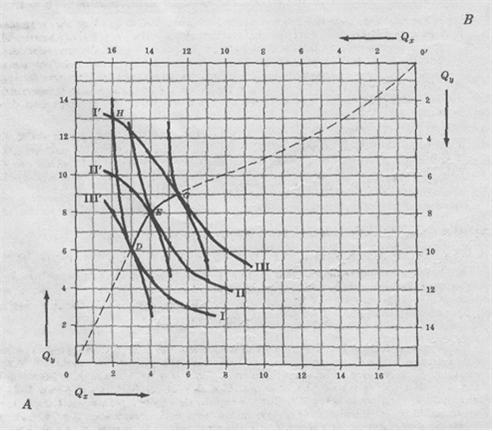In this diagram is called the Edgeworth box.

(b) Section H indicates that individual A has 13Y and 2X while individual B has 3Y 16X. At point H, the TMgSxy of A is higher than that of B. This means that A is willing to hand over and what is necessary to induce B to give a unit of X. Therefore, there is no basis for a mutually beneficial exchange in which to give up something to change her And that B will deliver X.

(c) a downward movement along the indifference curve I 'from the member center point G H to B leaves the individual at the same level of satisfaction, but places the individual A in the indifference curve III. Moreover, a downward movement along the curve I from H to D, left to individual A at the same level of satisfaction, but puts B on indifference curve III '. Since it is voluntary exchange, A and B will end somewhere between G and D (for example, point E on indifference curves II and II '), which implies that both individuals stand to gain from voluntary exchange. Note that the mutually beneficial exchange will end when one of the indifference curves of A is tangent to one of B, because at such points the TMgSxy of A is equal to B, points of contact as these are insured by the fact that the fields of indifference curves are dense.

17 .- (a) How can get the total contract curve in the case we discussed?
(b) What curve shows a contract?
(c) Show that the prerequisite for mutually beneficial exchange found in the analysis of utility is equivalent to that explained in the theory of indifference curves.

(a) The line connecting point D to E and G gives a contractual part of the curve for A and B. Drawing many indifference curves for A and B and uniting all points of contact, we could obtain the total contract curve. Such a curve extending from point 0 to point 0 'and would be analogous to the dotted line.

(b) Any point not on the curve indicates contractual basis for a mutually beneficial exchange. Once individuals are in the contract curve, can not get more gains from trade and commerce comes to an end. The greater the bargaining power of A in relation to that of B, the more it will approach the point A to G in the contract curve, and the greater the proportion of profit which A receives in exchange. The greater the bargaining power of B, the more it will come to approach the point D of the contract curve, and the greater the proportion of income received.

(c) The utility analysis, we found that the condition was UMgx / UMgy to A? UMgx / UMgy for B. In this chapter we have found no basis for mutually beneficial exchange if TMgSxy of A? TMgSxy B. However, we found that UMgx / UMgy = TMgSxy. We can therefore say that the exchange can take place if the UMgx / UMgy (TMgSxy) for A? UMgx / UMgy (= TMgSxy) for B.

INCOME-CONSUMPTION CURVE AND THE ENGEL CURVE

18.Si indifference curves I, Il and III of Problem 1 are consumer tastes (which remain unchanged during the analysis period = ceteris paribus) if the price of Y and X price remain unchanged at \$ 1 and \$ 2 respectively, and if the consumer's money income rises from \$ 12 to \$ 16 and then \$ 20 per time period, derive income-consumption curve and the Engel curve for this consumer.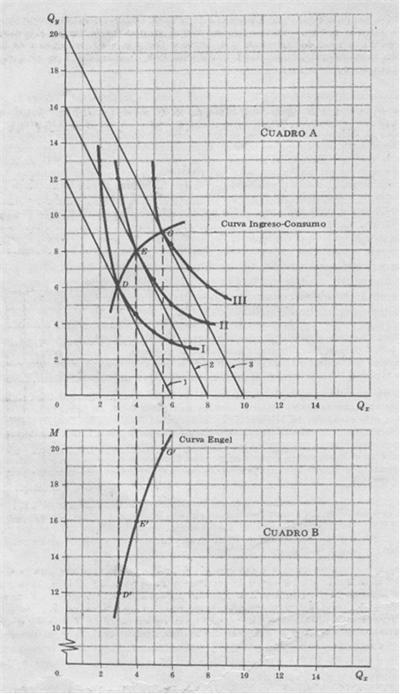Table A, the budget lines I, II and III are parallel to each other because Px / Py remains unchanged (at a value of 2). When consumer income is \$ 12 per time period, the consumer reaches its equilibrium at point D on indifference curve I buying 3X and 6Y. With an income of \$ 16, the consumer reaches equilibrium at point E on indifference curve II 4X buying and 8Y. With an income of \$ 20 per time period, the consumer reaches equilibrium at point G of the indifference curve III buying 5X and 9Y. SDR line joining the equilibrium of the consumer at various income levels and is a part. the income-consumption curve (ICC) for the consumer. Although SDR line was also part of the consumer contract curve in Diagram Edgeworth, this is just a coincidence and not necessarily so.)
UMgx Px
We note that in D, E, G of Table A the TMgSxy = --------- = ------ = 2
Py UMgy

We see that when we move from a consumer point of equilibrium to another, UMgx as UMgy can download, upload or remain unchanged. All that is needed for reasons of balance is that the reason for the UMgx to UMgy remains constant and equal to the TMgSxy and Px / Py.

Table B D'E'G line 'is part of the Engel curve of the consumer for product X. It shows that an income level of \$ 12 per time period, the consumer buys 3 units of X, the income level of \$ 16, purchase 4X, and the income level of \$ 20 purchase 5.5 units of X. Since the Engel curve for product X has a positive slope, m is positive and X is a normal product.

19 .- Based on the amount of income over the following Table,
(a) Draw the Engel curve
(b) To determine if this product is a necessity, a luxury or an inferior product at points A, B, D, F, M and L.

PuntoABCDFGHLIngreso (\$ / year) 4000600080001000012000140001600018000 Amount (kls. / year) 100200300350380390350250
(a)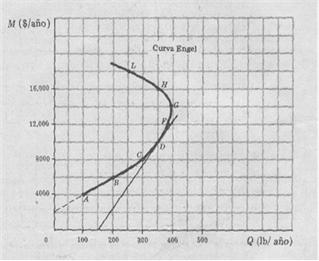(b) The tangent to the Engel curve at points A and B is positively sloped and cuts the axis of revenue, so the income elasticity of demand is greater than 1 and the product is generally a luxury in those stretches of income. In D and F, the slope of the tangent to the Engel curve is positive but short the quantity axis. Therefore, the income elasticity of demand is greater than 0 but less than 1, and the product is a necessity in these points (in these income levels). At points H and L, the Engel curve has negative slope which indicates that the product is inferior.
CURVE-CONSUMER PRICE AND CONSUMER DEMAND CURVE

20.-Suppose that the consumer equilibrium point is given in Problem 10, the price of X falls below \$ 2 per unit to \$ 1.
(a) Find the new equilibrium point, plot the price consumption curve of the consumer for the product X, and derive dx
(b) Is dx with respect to price elastic, inelastic or unitary elastic over this range of prices?
(c) Does not necessarily imply a TMgSxy decreasing UMgx and UMgy. decreasing? Is it essential for dx UMgx have decreasing negative slope?

a) Table A, point E is the original consumer equilibrium in Problem 10. When the price of X falls from \$ 2 to \$ 1 (ceteris paribus), we get the budget line 4 and a new consumer equilibrium point (point J on the indifference curve IV). Connecting points E and J, we obtain a curve segment of the consumer price consumption (CPC) for product X. From the consumer point of equilibrium in Table A, we can derive a segment dx, (Table B).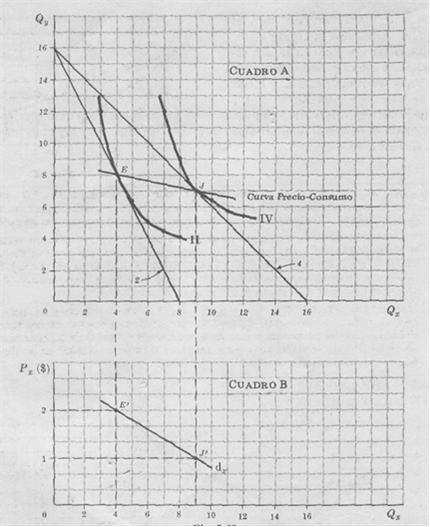(b) Since the CPC has a negative slope, dx is elastic with respect to price over the arch E'J '5 3 15 (a =- --- x --- = ---- = 1.15; -1 13 13 elsewhere. when the price of X falls from \$ 2 to \$ 1 on consumer spending increases for X \$ 8 to \$ 9 per time period, so that dx is price elastic over the arch E'J ').(c) In Section E of Table A, TMgSxy = UMgx / UMgy = 2. In Section J, TMgSxy = UMgx / UMgy = 1. Thus, in moving from point E to point J, the TMgSxy and the reason for the UMgy down UMgx. However, to lower the UMgx and need not UMgy the UMgx and UMgy decrease. For example, UMgy / UMgx may decrease even though these two quantities increase, provided that the increase is less than the UMgx the UMgy. Therefore, a growing TMgSxy not necessarily imply a decrease in UMgx and UMgy. Similarly, UMG is not needed to derive a downward sloping demand curve negatively.
21.En the following graph, the vertical axis measures the consumer's money income, while the horizontal X measures the amounts of the individual purchase period. Points C, D and E refer to different points of balance that results when only the price varies from X.
(a) What would show an indifference curve drawn in this system of axes?
(b) What does the price of X for a rotation in the direction of clockwise, the budget line 1 budget line 2, then the budget line 3?
(c) What kind of demand curve can be derived from the equilibrium points C, D and E?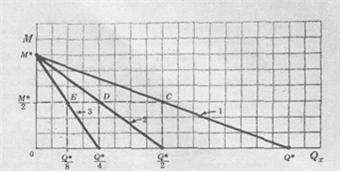(a) An indifference curve drawn on the system of axes show different combinations of money (not spent on X and therefore available to buy other products) and the quantity of X purchased which would equal customer satisfaction.
(b) A rotation in the sense of clockwise, the budget line 1 to 2 implies that the price of X is doubled (since consumer spending all your money in X could only buy exactly half X amount before buying). Similarly, a rotation in the direction of the clockwise heading 2 heading 3 implies that the price of X has doubled again.

(c) Joining the points C, D and E, we obtain the CPC, this consumer for product X. Since the CPC is horizontal, dx would have a unitary price elasticity on the arc has been defined. This is because as the price of X increases, the consumer buys fewer units of this product but still spending the same amount of income to buy it (exactly half that before).

SEPARATION OF EFFECTS OF SUBSTITUTION AND INCOME

22. Table A is equal to that of Example 11, with the difference K'J budget line '. Table B is derived from Table A and is equal to that of Example 12, except d'x
(a) How was K'J budget line "What does this line?
(b) What does a movement from point E to point G in Table A? A movement from G to T?
(c) How was dx in Table B? What shows?

(a) Heading K'J 'of CABLE A was obtained by shifting the budget line downward and KJ in a direction parallel to itself until it became tangent to the indifference curve II. Moving down the budget line KJ, reduced consumer money income. Moving down to the KJ line until it touches the indifference curve II, reduced consumer money income just enough to keep real income constant. (Note that according to this technique, the real income of consumers is constant if it reaches the same indifference curve, before and after the price change. In the example discussed here, the consumer money income must be reduced to \$ 3 their real income remains constant.) KJ budget line moves into position parallel to itself in order to keep the price of X relative to Y equal to 1 on the line K'J ', than it was in the KJ line.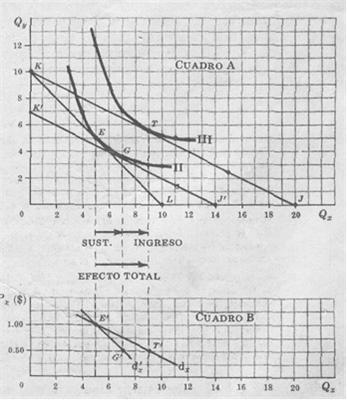(b) The movement from point E (on indifference curve II) to point G (same curve) represents the substitution effect of a change in price. The movement from point G (in the indifference curve II) to the point T (the indifference curve III) is the income effect is due to price change. We then have, for a given price change, total effect = Substitution effect Income effect + EG + ET = GT (c) Table B, d'x shows only the substitution effect of price change. So d'x, is the consumer demand curve for product X when real income (non-monetary income) consumers remains constant. Some economists prefer this type of demand curve, ie d'x, a the current demand curve constant money income. Unless otherwise specified, the term "demand curve" we always refer to the traditional or usual curve of demand. The technique to separate the income effect of the substitution effect is shown in Table A is useful not only to derive a demand curve along which real income remains constant, but also (which is perhaps more important) because it is a useful technique for analyzing many problems of great economic importance.
23.-From the graph of Problem 20
(a) separating the substitution effect resulting from a decrease in the price of X from \$ 2 to \$ 1 per unit (ceteris paribus),
(b) derive the consumer demand curve for product X when real income is held constant,
(c) with reference to the figure of the parts (a) and (b) explain how they derived the demand curve for product X along which money income is held constant, and
(d) explain how they derived the demand curve for product X along which real income remains constant.
(a) (b)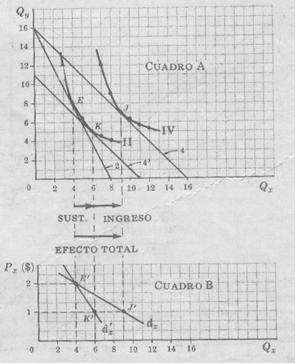(c) Table A, the consumer moves the equilibrium point E (on the line 2 and indifference curve II) to the equilibrium point J (budget line indifference curve 4 and IV) as a result of Px decreased, ceteris paribus. So when Px =\$ 2, the consumer buys 4X per unit time (point E 'in Schedule B dx), where Px = \$ 1, the consumer buys 9X (point Y in dx). Of the total increase in the amount of X demanded, partly due to substitution effects and the remainder to income effect. (As in Problem 18 we find that X is a normal product, both effects have the same direction.) Therefore, lowering Px (and move along down dx) consumer money income remains constant but its real income increases. It should be noted that in this example the substitution effect is greater than the income. In real life, the substitution effect is generally much stronger than the income effect. (D) Many economists prefer to keep constant the real income of consumers to derive a demand curve. One way to accomplish this is to reduce consumer money income enough to eliminate the income effect of price change. In the box this is achieved by moving the budget line 4 (parallel to itself) until it touches the curve indiferencia II. What we get is heading 4 'which reflects the same relative prices that line 4, but less than \$ 5 cash income. The movement from E to K along the indifference curve II is then the substitution effect of price change. The movement of K to J is the income effect of price change, d'x reflects only the substitution effect. Therefore, along d'x consumer's real income is held constant. Note that the price elasticity d'x, is smaller than dx.
24.-Beginning of a consumer equilibrium position
(a) separate the effect of replacing the income from a rise Depreciation (ceteris paribus) to a normal product,
(b) derive two demand curves for the product, one that remains constant money income and the other to keep constant the real income
(c) with reference to the figure of the parts (a) and (b) explain how they derived the demand curve for product X along which money income is constant, and
(d) explain how they derived the demand curve for product X along which money income is constant.
(a)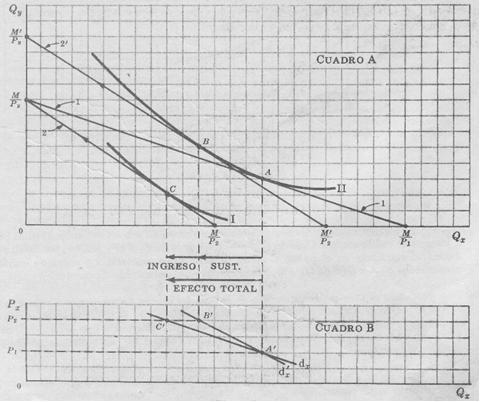(c) Table A, the consumer is originally in equilibrium at point A of the budget line indifference curve 1 and II. This gives the point A 'on yd'x dx, Table B. When the price of X increases from P 1 to P 2 (ceteris paribus), the consumer is in equilibrium at point C on the line 2 and indifference curve I. This gives the point C in dx, dx is the usual demand curve along which money income is held constant. The movement from A to C is the total effect of price change. Since the product is normal, the effect of income replacement and reinforce each other to reduce the amount of product to be price per period demand when its price rises.

(d) To derive d'x we have to isolate the income effect of price change. We do this by moving up the budget line 2 parallel to itself until it is tangent to the indifference curve II. This gives us the budget line 2 '. This shift from line 2 to 2 'corresponds to an increase in money income consumers from M to M', maintaining the same relative prices of goods given by the slope of the budget line 2. Heading 2 'is tangent to the indifference curve II at point B. The movement along the indifference curve I from A to B represents the substitution effect of price gouging. Elmovimiento from B to C refers to the income effect, d'x only shows the substitution effect of price change. Therefore, along d'x, remains constant real income. Note that the price elasticity of d'x is less than dx.

25.-Beginning with the equilibrium position A consumer in the graph, determine (a) The overall effect of reducing P Px2 to P 1,
(b) The effect of substitution and
(c) the income effect.
(d) What kind of product is X?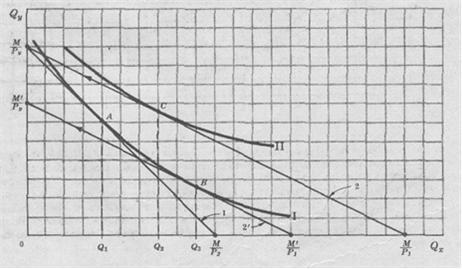(a) In the figure, the consumer is originally in equilibrium at point A budget line indifference curve 1 and I. When the price of low X P2 to P1 (ceteris paribus), the consumer will come to equilibrium at point C on the budget line 2 and indifference curve II. The movement from A to C (or from Q1 to Q2) is the total effect of price change.
(b) To find the substitution effect of the low price, we must eliminate the effect of the total effect of income. This is achieved by moving down the budget line 2 budget line 2 '. The latter is tangent to the indifference curve at point B. I The movement along the indifference curve I from A to B (which is equal to Q1 Q3) is the substitution effect of price change.

(c) Since the substitution effect (Q1 Q3) exceeds the total effect (Q1 Q2), the income effect should be opposite to that of substitution. The income effect is given by a movement from B to C and equals Q3Q2

(d) In this case, the income effect is opposite of the substitution effect. Therefore, X is an inferior product, but not a Giffen product because, when the price of X, the quantity demanded per period of time increases from Q1 to Q2 (the total effect). Note that in this case the demand curve along which real income is constant would have a price elasticity greater than the demand curve along which remained constant money income. However, both demand curves slope downward.

26 .- Starting with a consumer equilibrium position,
(a) show the effect of substitution and income effect of a low price for a product Giffen.
(b) Does sloping demand curve for a Giffen product along which money income is constant? Why?
(a)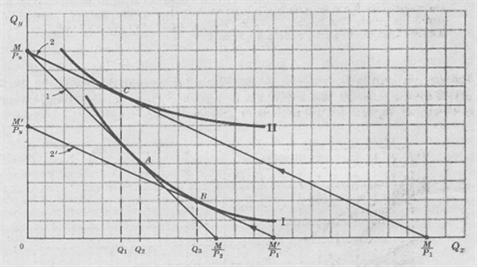Point A is the original point of equilibrium. When the price of low X P2 to P1 (ceteris paribus), the consumer is in equilibrium at point C. The movement from A to C (or Q2 to Q1) is the total effect of price change. Therefore, X is a Giffen product since the lower the price consumers buy less. The movement from A to B (or from Q2 to Q3) is the substitution effect of price change. For the total effect is negative, the income effect has to be not only contrary to the substitution but has to be very superior to him. This happens very rarely, if ever. In the figure, the income effect is given by a movement from B to C (or Q3 to Q1).

(b) Since this is a Giffen product, the demand curve along which is constant money income has a positive slope, but the demand curve along which real income is constant slopes negative. This is because the substitution effect always leads to an increase in the quantity demanded of a product when its price falls, whatever the type of product concerned.

SOME CONSIDERATIONS AND APPLICATIONS

27. (A) What relationship exists between the views of the utility and indifference curves in the theory of consumer demand?
(b) What is the basic difference between these two views?
(c) Which is preferable? Why?

(a) The study of consumer demand, theory of indifference curves can be used as an alternative to the previous utility theory in order to analyze consumer behavior (eg, balance and exchange) , and derive a consumer demand curve for a product.

(b) The basic difference between the theories of utility and indifference curves, is that the former rests on the assumption stronger and a bit unreal that utility is measurable in cardinal direction, while the theory of curves indifference requires only ordinal measure of utility or satisfaction. In other words, the theory of indifference curves only requires the consumer to be able to decide if a basket of goods gives greater satisfaction, equal, or less than the satisfaction that give other baskets of goods, without requiring allocated to each basket a number of utils.

(c) Many economists prefer the theory of indifference curves to the utility, because it requires only an ordinal measure (rather than cardinal) utility, and also because it allows us to separate, more easily than the theory of utility, the effect of replacing the income effect of a price change.

28.Supongamos a poor family "typical" is in equilibrium at point A 1 and the budget line indifference curve I of the chart. (At this point, the family spends \$ 1,000 of their income from \$ 5,000 to buy 100 units of food.) Suppose now the government decides to raise the level of utility or satisfaction of this family, the indifference curve I to the indifference curve II.
(a) How can the government achieve this objective by subsidizing food consumption of the family (ie, paying part of the price of food).
(b) What would the cost of this program to the government for each typical poor family. (c) What else could the government get the same result? At what cost? (d) What reasons would prompt the government to choose anyway the most expensive program to achieve the results you want?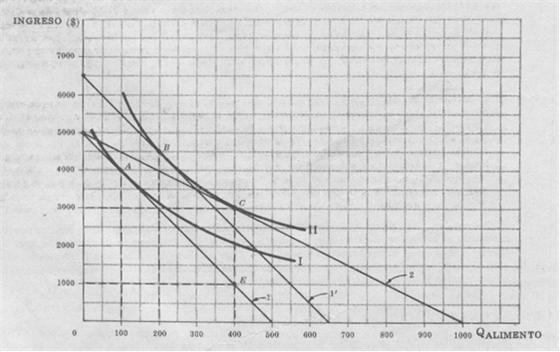(a) One way the government can raise the level of utility or satisfaction of this poor family from point A on the line 1 and indifference curve I to the indifference curve II, is to let her buy food at half the price market, paying the government the other half of the market price. With this subsidy to food consumption, this poor family would reach a new equilibrium at point C on the budget line 2 and indifference curve II.

(b) To reach the point C in the previous program, this poor family spends \$ 2,000 of their income to buy 400 units of food (see chart). Without the subsidy, the family would have had to spend \$ 4,000 to buy those 400 units (point E in figure). It is seen that the cost to the government program would be \$ 2,000 (EC) for poor families 'typical'.

(c) The Government may also make its goal of raising the level of satisfaction of this poor family of the indifference curve I to the indifference curve II, paying a cash grant of \$ 1,500. With this cash assistance, the family would be in equilibrium at point B of the budget line 1 'and indifference curve II. At point B, the family spends \$ 2,000 of income (\$ 6,500) to buy 200 units of food (see chart).

(d) While the first program is more expensive, the government may however prefer horn because it has resulted in increased food consumption by the family. This increased food consumption may itself be one of the objectives pursued by the government with its aid program.

29.-Suppose that a person spends all his income in just three products: X, Y and Z. Assume further that we know that X and Y are normal goods and that X is a surrogate (substitute) of Y (in the sense that if the individual consumes more than X has to consume less and to remain at the same level of satisfaction ie in the same indifference curve.)
(a) Explain how to operate the effects of substitution and income when the price of X (ceteris paribus).

(b) Under what conditions the sign is negative and x (even though we know that X and Y are substitutes)?

(a) As the price of X increases the quantity demanded by this individual per unit time, because of substitution effects and income. This is reflected in a downward movement along the demand curve for X, which has negative slope. However, when the price of X, we have two opposing forces affecting the demand for Y. The substitution effect, by itself, tends to reduce the demand for Y because X is a substitute for Y. The effect of income, by itself, tends to increase demand for Y because Y (same as X) is a normal product. If the substitution effect exceeds the income effect (as is usual), and low demand (ie, d moves down) and x, has the sign "right" or negative.

(b) If the income effect is stronger than the opposite substitution effect, d will shift up when the price of X. This is possible though rare. In this case, and x has the sign "wrong" (that is, and x will be negative which would indicate that X and Y are complementary, although we know that X and Y are actually substitutes (substitutes) from each other).

Theoretically there is a more accurate method to rely on the sign of the cross-elasticity to determine whether goods are substitutes or complements. But that method, not more complicated, not very useful from a practical standpoint. In empirical work, the method of cross-elasticity is what is typically used to classify the type of relationship between the goods, although it can sometimes lead to one conclusion dirty.